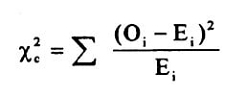# Chi-Square Test

## Introduction to the Chi-Square Test & how it is performed

A chi-squared test is written as the χ² test, which is a statistical analysis hypothesis testing method. The basic idea behind this test is to examine whether your data is as expected or not. This Chi-square test compares the observed values in your data to the expected values to check if the null hypothesis is true. This article provides a scope to understand Chi-square distribution in a data set to analyse the significance level and degree of freedom.

## How is the Chi-Square test performed?

This Chi-Square statistic is commonly utilised to test the interrelationships between the categorical variables. The null hypothesis of the Chi-Square test refers to that, no relationship exists on the categorical variables in the population; and they are independent of each other. There are mainly three types of Chi-square tests, which are tests of goodness of fit, independence and homogeneity. Chi-square is non-symmetric, where two non-negative integers are considered to explore the value of Chi-square. There are many different chi-square distributions, one for each degree of freedom, and for example, if the population variance is one, n=1.

1. The formula of chi-square is,1. Where,
2. X2 is chi square
3. Oi is observed value
4. Ei is expected value

The Chi-square goodness of fit test is suitable to identify whether the sample data matches the population or not. On the other hand, the Chi-square test for independence indicates the comparison between two variables in contingency tables to see they are related or not. Hence, through this chi-square test, it is possible to analyse the distribution of the categorical variables that differs from each other. The p-value can be explored through the Chi-square test where it is helpful to analyse the test result is significant or not. The alpha level (α) is chosen by the researchers where the level is 0.05 (5%), and it has the levels like 0.01 or 0.10.

## Uses of Chi-square

1. In a normal distribution set, the sample standard deviation is being calculated to have confidence interval estimation for the articular population standard deviation
2. The relationship between the categorical values can also be calculated through this test.
3. The goodness of fit, as well as standard variance in the sample through normal distribution, is also possible to be measured through the Chi-square test.
4. Tests of deviations of differences between expected and observed frequencies (one-way tables) are performed well.
5. The purpose of the chi-square test is to determine if a difference between observed data and expected data is due to chance, or if it is due to a relationship between the existing variables in the data set.

Hence, the chi-square test is mainly calculated by evaluating the cell frequencies which is involved with the expected frequencies in those types of cases, when there is no association between the variables. Through the comparison between the expected type of frequency and the actual observed frequency is then made in this test, it is also possible to analyse the independent variables in the data set.

It has been explored that, Chi-square statistical consulting measure for the researchers to analyse the differences between the observed values and expected values. The quantitative data analysis is mandatory to gather authentic data directly from the respondents and it will further provide an opportunity to the researchers to calculate the variances in expected and observed values. The significance level of the independent variable can also be evaluated further through this chi-square statistical test. Hereby, for critical evaluation of the independent and dependant data in the data set, it is helpful for the researchers to utilise the chi-square test to explore the p-value of 0.05 or greater for testing the final hypothesis.

Chi-square tests can be run on either Microsoft Excel or Google Sheets, we at SPSS-Tutor experts help you with software packages too that are more intuitive to run the test.